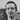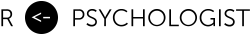# Power analysis for longitudinal multilevel models: powerlmm 0.2.0 is now out on CRAN

March 21, 2018

My R packge powerlmm 0.2.0 is now out on CRAN. It can be installed from CRAN https://cran.r-project.org/package=powerlmm or GitHub https://github.com/rpsychologist/powerlmm.

# Changes in version 0.2.0

## New features

• Analytical power calculations now support using Satterthwaite’s degrees of freedom approximation.
• Simulate.plcp will now automatically create lme4 formulas if none is supplied, see ?create_lmer_formula.
• You can now choose what alpha level to use.
• Treat cluster sizes as a random variable, uneqal_clusters now accepts a function indicating the distribution of cluster sizes, via the new argument func, e.g. rpois or rnorm could be used to draw cluster sizes.
• Expected power for designs with parameters that are random variables, can be calculated by averaging over multiple realizations, using the argument R.
• Support for parallel computations on Microsoft Windows, and in GUIs/interactive environments, using parallel::makeCluster (PSOCK). Forking is still used for non-interactive Unix environments.

## Improvements

• Calculations of the variance of the treatment effect is now much faster for designs with unequal clusters and/or missing data, when cluster sizes are large. The calculations now use the much faster implementation used by lme4.
• Cleaner print-methods for plcp_multi-objects.
• Multiple power calculations can no be performed in parallel, via the argument cores.
• simulate.plcp_multi now have more options for saving intermediate results.
• print.plcp_multi_power now has better support for subsetting via either [], head(), or subset().

## Breaking changes

• icc_pre_subject is now defined as (u_0^2 + v_0^2) / (u_0^2 + v_0^2 + error^2), instead of (u_0^2) / (u_0^2 + v_0^2 + error^2). This would be the subject-level ICC, if there’s no random slopes, i.e. correlation between time points for the same subject.
• study_parameters(): 0 and NA now means different things. If 0 is passed, the parameters is kept in the model, if you want to remove it specify it as NA instead.
• study_parameters(): is now less flexible, but more robust. Previously a large combination if raw and relative parameters could be combined, and the individual parameters was solved for. To make the function less bug prone and easier to maintain, it is now only possible to specify the cluster-level variance components as relative values, if the other parameters as passed as raw inputs.

## Bug fixes and minor changes

• Output from simulate_data() now includes a column y_c that contains the full outcome vector, without missing values added. This makes it easy to compare the complete and incomplete data set, e.g. via simulate().
• simulate() new argument batch_progress enables showing progress when doing multiple simulations.
• Fix bug in summary.plcp_sim where the wrong % convergence was calculated.
• Simulation function now accepts lme4 formulas containing ”||“.
• The cluster-level intercept variance is now also set to zero in the control group, when a partially nested design is requested.
• Fix incorrect error message from study_parameters when icc_cluster_pre = NULL and all inputs are standardized.
• Fix bug that would cause all slopes to be zero when var_ratio argument was passed a vector of values including a 0, e.g. var_ratio = c(0, 0.1, 0.2).
• Fix bug for multi-sim objects that caused the wrong class the be used for, e.g. res[]\$paras, and thus the single simulation would not print correctly.
• Results from multi-sim objects can now be summarized for all random effects in the model.
• More support for summarizing random effects from partially nested formulas, e.g. cluster_intercept and cluster_slope is now correctly extracted from (0 + treatment + treatment:time || cluster).
• When Satterthwaite’s method fails the between clusters/subjects DFs are used to calculate p-values.
• Power.plcp_multi is now exported.
• get_power.plcp_multi now shows a progress bar.
• Fix a bug that caused dropout to be wrong when one condition had 0 dropout, and deterministic_dropout = FALSE.Written by Kristoffer Magnusson a researcher in clinical psychology. You should follow him on Twitter and come hang out on the open science discord Git Gud Science.

Share:

Published March 21, 2018 (View on GitHub)